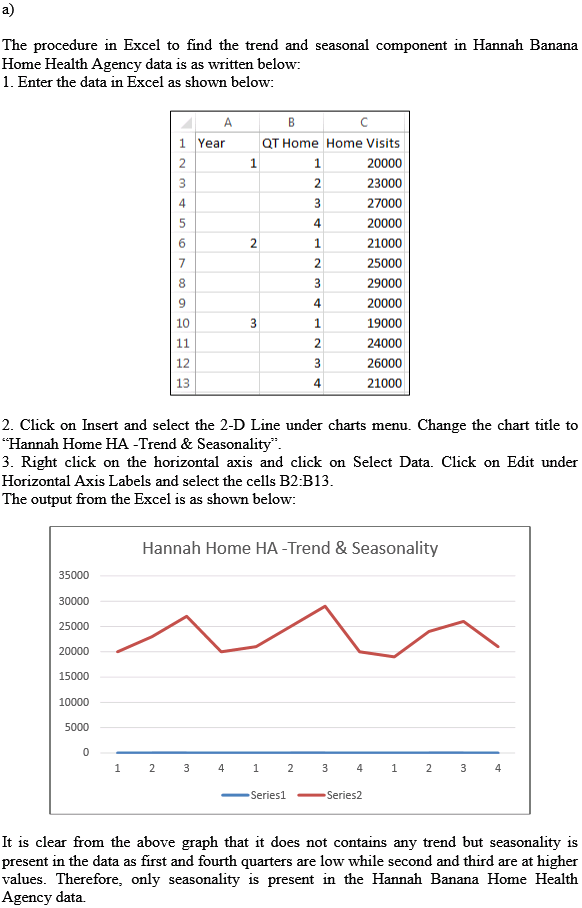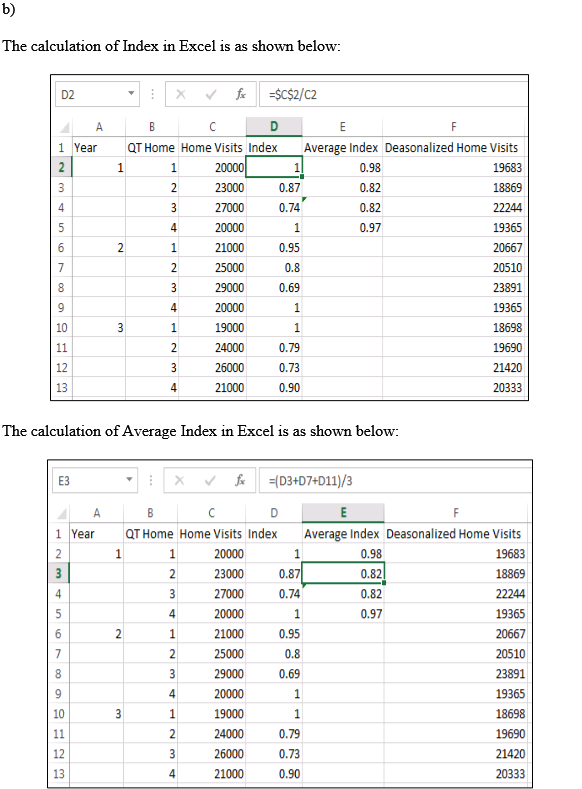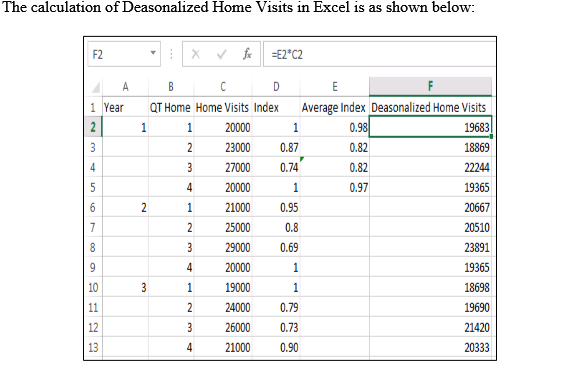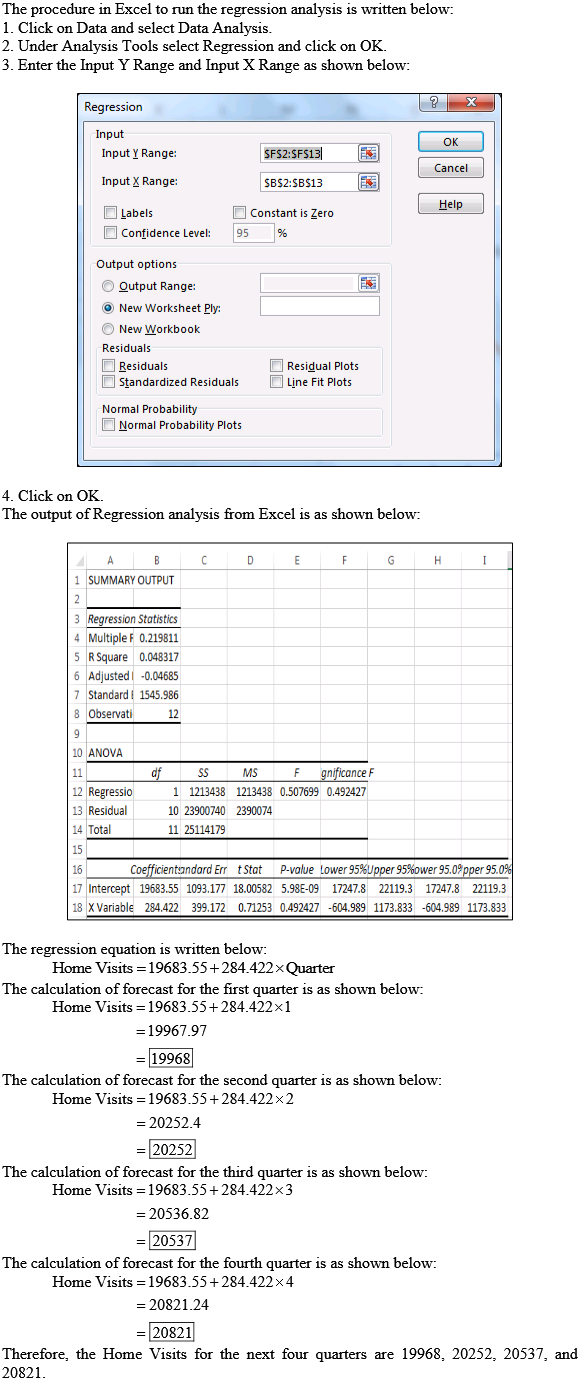# Quiz 7: Predicting Future Costs

Business

Regression analysis is the statistical process of predicting the relationships among variables. Linear regression refers to the most basic and commonly used estimation technique or a predictive technique. The primary difference between simple and multiple regressions is written as below: Only one independent variable is used by the simple regression to predict the dependent variable. But in multiple regressions two or more than two independent variables are used to predict the dependent variable.The cost estimation and prediction of future cost are still difficult even after adjusting historical data with impact of inflation as some of the costs are mixed cost. To make reasonably good prediction of future cost it is important to estimate the portion of fixed cost and variable cost from mixed cost to certain extent. The techniques for estimating such costs are High-Low Technique and Linear Regression. High-Low Technique High-Low technique is one of the methods which is utilized to split the cost into variable and fixed component. It is easy to understand but is not reliable. It analyzes the historical cost data, and then selects the highest and lowest volume and their respective cost. The cost difference is divided by the change in volume which results in change in cost per unit with change in volume. Linear Regression Under Regression method, all the data are considered which are used to estimate volume which is more precise and accurate than calculated in high and low technique. Here, Volume and cost to predict a line of fit which gives better estimate of the future. It is not possible for a straight line to go through all the historical data/point as they are slightly scattered, but it helps to identify line where it is close to historical data/point. Advantages of regression methods: The major drawback of high low technique is that it deals only with the extremes value which means that highest and lowest value from the entire data which makes it less reliable. This drawback is avoided by using regression method. Regression method uses entire data therefore any change in cost behavior will have less impact of estimations. Method of regression is more reliable. Method of regression enables manager to establish a relation between dependent and independent variable thus helping in decision making. The Linear regression consider historical information about volume and cost and try to establish a single line which can give reasonably good estimates as it uses independent variable (i.e volume) to predict dependent variable (i.e costs). Therefore with available data for last five years of total utility cost and total meal a line can be drawn which is close to point of last five years utility cost which will provide better estimate of future. However the estimate may not be accurate but the possibility of substantial difference between the prediction and actual results will be low. Thus, it can be concluded that method of regression can be used for better forecast.